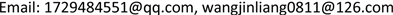﻿ 记忆依赖微分方程组解的存在唯一性 The Existence and Uniqueness of Solution for Memory-Dependent Differential Equations

Vol. 09  No. 02 ( 2020 ), Article ID: 34224 , 8 pages
10.12677/AAM.2020.92022

The Existence and Uniqueness of Solution for Memory-Dependent Differential Equations

Xia Liu, Jinliang Wang

Department of Mathematics, Qingdao Technological University, Qingdao ShandongReceived: Jan. 30th, 2020; accepted: Feb. 12th, 2020; published: Feb. 19th, 2020ABSTRACT

The weighted function of the memory dependent derivative can be selected according to the actual situation compared with the fractional derivative, which has stronger expressiveness. This paper mainly discusses the existence and uniqueness of the solution of the first-order memory dependent differential equations. Find the exact solution of the first-order linear memory dependent differential equations in special cases. Firstly, a series of transformations are performed by using the partial integral-pair equation, and then the vector series convergence is further demonstrated by constructing the Picard iteration sequence, which proves that the solution of the equation system exists when its delay is small enough and the weighted function is first-order differentiable; and then the solution is demonstrated uniquely by the Grownwall inequality. Find the exact solution of the first-order linear memory dependent differential equations when the weighted function takes a specific form. By comparing it with the solution of the ordinary differential equations, it is found that the solution is more and more close when the delay is larger.

Keywords:Memory Dependent Derivative, The Fractional Derivative, Uniqueness, Existence, The Picard Iteration1. 引言

${D}_{\tau }^{m}f\left(t\right)=\frac{1}{\tau }{\int }_{t-\tau }^{t}\text{ }K\left(t-s\right){f}^{m}\left(s\right)\text{d}s.$ (1)

${D}_{\tau }^{m}f\left(t\right)=\frac{1}{R}{\int }_{t-\tau }^{t}\text{ }K\left(t-s\right){f}^{m}\left(s\right)\text{d}s.$ (2)

$R={\int }_{t-\tau }^{t}\text{ }K\left(t-s\right)\text{d}s.$

$t-s=r$，则有

$R={\int }_{0}^{\tau }\text{ }K\left(r\right)\text{d}r.$

$\left(\begin{array}{l}{D}_{\tau }U=F\left(t,U\right),0 (3)

$‖F\left(t,U\right)‖=\sqrt{{f}_{1}{\left(t,u,v\right)}^{2}+{f}_{2}{\left(t,u,v\right)}^{2}},$

$F\left(t,U\right)$ 满足范数下的Lipschiz条件即存在 $L>0$，使得

$‖F\left(t,{U}_{2}\right)-F\left(t,{U}_{1}\right)‖\le L‖{U}_{2}\left(t\right)-{U}_{1}\left(t\right)‖,0\le t\le h.$

${D}_{\tau }U=\frac{1}{R}{\int }_{t-\tau }^{t}\text{ }K\left(t-s\right)\frac{\partial U}{\partial s}\text{d}s=\frac{1}{R}U\left(t\right)-\frac{1}{R}{\int }_{t-\tau }^{t}\frac{\partial K}{\partial s}U\left(s\right)\text{d}s.$ (4)

$U\left(t\right)={\int }_{t-\tau }^{t}\text{ }{\partial }_{s}K\cdot U\left(s\right)\left(s\right)\text{d}s+\tau R\cdot F\left(t,U\right).$ (5)

$U\left(t\right)={\int }_{0}^{t}\text{ }{\partial }_{s}K\cdot U\left(s\right)\text{d}s+{\int }_{t-\tau }^{0}\text{ }{\partial }_{s}K\cdot {U}^{*}\left(s\right)\text{d}s+\tau R\cdot F\left(t,U\right).$ (6)

$M=\underset{\left(t-s\right)\in \left[0,\tau \right]}{max}|\partial K/\partial s|.$

${U}_{0}\left(t\right)={U}^{*}\left(0\right),t\in \left[0,h\right],$ (7)

${U}_{n}\left(t\right)={\int }_{0}^{t}\text{ }\text{ }{\partial }_{s}K\cdot {U}_{n-1}\left(s\right)\text{d}s+{\int }_{t-\tau }^{0}\text{ }\text{ }{\partial }_{s}K\cdot {U}^{*}\left(s\right)\text{d}s+\tau R\cdot F\left(t,{U}_{n-1}\left(t\right)\right),$ (8)

${U}_{0}\left(t\right)+\underset{k=1}{\overset{\infty }{\sum }}\left[{U}_{k}\left(t\right)-{U}_{k-1}\left(t\right)\right],0\le t\le h$ (9)

$\begin{array}{c}‖{U}_{2}\left(t\right)-{U}_{1}\left(t\right)‖\le M{\int }_{0}^{t}‖{U}_{1}\left(s\right)-{U}_{0}\left(s\right)‖\text{d}s+\tau RL‖{U}_{1}\left(t\right)-{U}_{0}\left(t\right)‖\\ \le \left(Mt+\tau RL\right)N\\ \le \left(M+RL\right)\tau N.\end{array}$

$‖{U}_{k}\left(t\right)-{U}_{k-1}\left(t\right)‖\le {\left(\left(M+LR\right)\tau \right)}^{k-1}N.$ (10)

$\begin{array}{l}‖{U}_{k+1}\left(t\right)-{U}_{k}\left(t\right)‖\\ \le M{\int }_{0}^{t}‖{U}_{k}\left(s\right)-{U}_{k-1}\left(s\right)‖\text{d}s+\tau RL‖{U}_{k}\left(t\right)-{U}_{k-1}\left(t\right)‖\\ \le {\left(\left(M+LR\right)\tau \right)}^{k}N.\end{array}$

$‖{U}_{n}\left(t\right)-{U}_{n-1}\left(t\right)‖\le {\left(\left(M+LR\right)\tau \right)}^{n-1}N.$ (11)

$\underset{k\to \infty }{lim}{U}_{k}\left(t\right)=U\left(t\right),\text{\hspace{0.17em}}0\le t\le h.$ (12)

$\underset{n\to \infty }{lim}{U}_{n}\left(t\right)=\underset{n\to \infty }{lim}\left\{{\int }_{0}^{t}\text{ }\text{ }{\partial }_{s}K\cdot {U}_{n-1}\left(s\right)\text{d}s+{\int }_{t-\tau }^{0}\text{ }\text{ }{\partial }_{s}K\cdot {U}^{*}\left(s\right)\text{d}s+\tau R\cdot F\left(t,{U}_{n-1}\left(t\right)\right)\right\}.$

$\underset{n\to \infty }{lim}{U}_{n}\left(t\right)={\int }_{0}^{t}\text{ }\text{ }{\partial }_{s}K\cdot \underset{n\to \infty }{lim}{U}_{n-1}\left(s\right)\text{d}s+{\int }_{t-\tau }^{0}\text{ }\text{ }{\partial }_{s}K\cdot {U}^{*}\left(s\right)\text{d}s+\tau R\cdot F\left(t,\underset{n\to \infty }{lim}{U}_{n-1}\left(t\right)\right).$

$U\left(t\right)={\int }_{0}^{t}\text{ }\text{ }{\partial }_{s}K\cdot U\left(s\right)\text{d}s+{\int }_{t-\tau }^{0}\text{ }\text{ }{\partial }_{s}K\cdot {U}^{*}\left(s\right)\text{d}s+\tau R\cdot F\left(t,U\left(t\right)\right),$

$‖W\left(t\right)-U\left(t\right)‖={\int }_{0}^{t}\text{ }\text{ }{\partial }_{s}K\cdot ‖W\left(s\right)-U\left(s\right)‖\text{d}s+\tau R\cdot ‖F\left(t,W\left(t\right)\right)-F\left(t,U\left(t\right)\right)‖.$

$‖W\left(t\right)-U\left(t\right)‖\le {\int }_{0}^{t}|{\partial }_{s}K|\cdot ‖W\left(s\right)-U\left(s\right)‖\text{d}s+\tau RL‖W\left(t\right)-U\left(t\right)‖.$ (13)

$\left(1-\tau RL\right)\cdot ‖W\left(t\right)-U\left(t\right)‖\le 0+{\int }_{0}^{t}|{\partial }_{s}K|\cdot ‖W\left(s\right)-U\left(s\right)‖\text{d}s.$ (14)

$0\le ‖W\left(t\right)-U\left(t\right)‖\le \frac{0}{1-\tau RL}\cdot exp\left\{{\int }_{0}^{t}|{\partial }_{s}K|/\left(1-\tau RL\right)\text{d}s\right\}=0.$

2. 线性方程组的解法

$\left(\begin{array}{l}{D}_{\tau }U=AU+F\left(t\right),0 (15)

${D}_{\tau }U=\frac{1}{\tau }{\int }_{t-\tau }^{t}\text{ }\text{ }K\left(t-s\right)\frac{\partial U}{\partial s}\text{d}s=\frac{1}{\tau }U\left(t\right)-\frac{1}{\tau }{\int }_{t-\tau }^{t}\frac{\partial K}{\partial s}U\left(s\right)\text{d}s.$

${D}_{\tau }U=\frac{1}{\tau }U\left(t\right)-\frac{1}{{\tau }^{2}}{\int }_{t-\tau }^{t}\text{ }U\left(s\right)\text{d}s,$ (16)

$U\left(t\right)=\frac{1}{\tau }{\int }_{t-\tau }^{t}{\left(E-A\tau \right)}^{-1}U\left(s\right)\text{d}s+\tau {\left(E-A\tau \right)}^{-1}F\left(t\right),$ (17)

${U}^{\prime }\left(t\right)=\frac{1}{\tau }{\left(E-A\tau \right)}^{-1}U\left(t\right)-\frac{1}{\tau }{\left(E-A\tau \right)}^{-1}{U}^{*}\left(t-\tau \right)+\tau {\left(E-A\tau \right)}^{-1}{F}^{\prime }\left(t\right).$ (18)

$\begin{array}{l}{u}^{\prime }\left(t\right)=\frac{e}{\tau }u\left(t\right)+\frac{f}{\tau }v\left(t\right)-\frac{e}{\tau }{u}^{*}\left(t-\tau \right)-\frac{f}{\tau }{v}^{*}\left(t-\tau \right)+e\tau {f}_{1}\left(t\right)+f\tau {f}_{2}\left(t\right),\\ {v}^{\prime }\left(t\right)=\frac{g}{\tau }u\left(t\right)+\frac{h}{\tau }v\left(t\right)-\frac{g}{\tau }{u}^{*}\left(t-\tau \right)-\frac{h}{\tau }{v}^{*}\left(t-\tau \right)+g\tau {f}_{1}\left(t\right)+h\tau {f}_{2}\left(t\right).\end{array}$ (19)

${D}_{\tau }U=\frac{1}{\tau }U\left(t\right)-\frac{n}{{\tau }^{2}}{\int }_{t-\tau }^{t}{\left(\frac{s-t}{\tau }+1\right)}^{n-1}U\left(s\right)\text{d}s,$

$U\left(t\right)=\frac{n}{\tau }{\int }_{t-\tau }^{t}{\left(\frac{s-t}{\tau }+1\right)}^{n-1}U\left(s\right)\text{d}s+\tau F\left(t\right).$ (20)

${U}^{\prime }\left(t\right)=-\frac{n\left(n-1\right)}{{\tau }^{2}}{\int }_{t-\tau }^{t}{\left(\frac{s-t}{\tau }+1\right)}^{n-2}U\left(s\right)\text{d}s+\frac{n}{{\tau }^{2}}U\left(t\right)-\frac{n}{{\tau }^{2}}{u}^{*}\left(t-\tau \right)+\tau {F}^{\prime }\left(t\right).$ (21)

$U\left(t\right)$ n阶可导时，对上式进行 $n-1$ 次求导，可以得到关于 $U\left(t\right)$ 的n阶微分方程，求解常微分方程组，即可得到(3)的解。

3. 与常微分方程组相比

$\left(\begin{array}{l}{D}_{\tau }U=AU+F\left(t\right),0 (22)

$U=\left[\begin{array}{c}u\\ v\end{array}\right],$ $A=\left[\begin{array}{cc}1/\tau & 2/\tau \\ 2/\tau & 1/\tau \end{array}\right],$ $F\left(t\right)=\left[\begin{array}{c}{\text{e}}^{3t/\tau }+{\text{e}}^{-t/\tau }\\ {\text{e}}^{-t/\tau }-{\text{e}}^{3t/\tau }\end{array}\right]$

$\left(\begin{array}{l}{u}^{*}\left(t\right)=3{\tau }^{\left(3t+3\tau \right)/\tau }+\tau {\text{e}}^{\left(-t-\tau \right)/\tau },-\tau \le t\le 0\\ {v}^{*}\left(t\right)=-3{\tau }^{\left(3t+3\tau \right)/\tau }-\tau {\text{e}}^{\left(-t-\tau \right)/\tau }.-\tau \le t\le 0\end{array}$

$\begin{array}{l}u=\left(3\tau {\text{e}}^{3}-\tau {\text{e}}^{-1}+{10}^{-4}\right){\text{e}}^{-t/\tau }-{10}^{-4}\cdot {\text{e}}^{t/\tau }.\\ v=\left(-3\tau {\text{e}}^{3}-\tau {\text{e}}^{-1}+{10}^{-4}\right){\text{e}}^{-t/\tau }-{10}^{-4}\cdot {\text{e}}^{t/\tau }.\end{array}$

$\left(\begin{array}{l}{U}^{\prime }\left(t\right)=AU+F\left(t\right),0 (23)

$\left(\begin{array}{l}{u}^{*}\left(0\right)=3{\tau }^{\left(3t+3\tau \right)/\tau }+\tau {\text{e}}^{\left(-t-\tau \right)/\tau }.\\ {v}^{*}\left(0\right)=-3{\tau }^{\left(3t+3\tau \right)/\tau }-\tau {\text{e}}^{\left(-t-\tau \right)/\tau }.\end{array}$

$\begin{array}{l}u\left(t\right)=\left(3\tau {\text{e}}^{3}-\tau {\text{e}}^{-1}+{10}^{-4}\right){\text{e}}^{-t/\tau }-{10}^{-4}\ast {\text{e}}^{3\ast t/\tau },\\ v\left(t\right)=\left(-3\tau {\text{e}}^{3}-\tau {\text{e}}^{-1}+{10}^{-4}\right){\text{e}}^{-t/\tau }-{10}^{-4}\ast {\text{e}}^{3\ast t/\tau }\end{array}$Figure 1. (a) (b) When $\tau =1$, memory dependent derivative differential equation with constant differential equation component u and component v; (c) (d) When $\tau =0.5$, memory dependent derivative differential equation with constant differential equation component u and component v

4. 结论

The Existence and Uniqueness of Solution for Memory-Dependent Differential Equations[J]. 应用数学进展, 2020, 09(02): 187-194. https://doi.org/10.12677/AAM.2020.92022

1. 1. Wang, J.L. and Li, H.F. (2011) Surpassing the Fractional Derivative: Concept of the Memory-Dependent Derivative. Computers and Mathematics with Applications, 62, 1562-1567. https://doi.org/10.1016/j.camwa.2011.04.028

2. 2. Sun, W.W. and Wang, J.L. (2017) On Numerical Solution of the Memory Dependent Partial Differential Equations. Advances in Applied Mathmatics, 6, 637-643. https://doi.org/10.12677/AAM.2017.64074

3. 3. Li, H.F. and Wang, J.L. (2012) Molding the Dynamic System with Memory-Dependent Derivative. 24th Chinese Control and Decision Conference (CCDC), Taiyuan, 23-25 May 2012, 1032-1036. https://doi.org/10.1109/CCDC.2012.6244162

4. 4. Sun, W.W. and Wang, J.L. (2018) Reconstruct the Heat Con-duction Model with Memory Dependent Derivative. Applied Mathematics, 9, 1072-1080. https://doi.org/10.4236/am.2018.99072

5. 5. Ezzat, M.A., El-Karamany, A.S. and El-Bary, A.A. (2016) General-ized Thermoelasticity with Memory-Dependent Derivatives Involving Two Temperatures. Mechanics of Advanced Ma-terials and Structures, 23, 545-553. https://doi.org/10.1080/15376494.2015.1007189

6. 6. Ezzat, M.A. and El-Bary, A.A. (2016) Thermoelectric MHD with Memory-Dependent Derivative Heat Transfer. International Communications in Heat and Mass Transfer, 75, 270-281. https://doi.org/10.1016/j.icheatmasstransfer.2016.04.026

7. 7. Ezzat, M.A. and El-Bary. A.A. (2015) Memory-Dependent Derivatives Theory of Thermo-Viscoelasticity Involving Two-Temperature. Journal of Mechanical Science and Technology, 29, 4273-4279. https://doi.org/10.1007/s12206-015-0924-1

8. 8. Shaw, S. (2019) Theory of Generalized Thermoelasticity with Memory-Dependent Derivatives. American Society of Civil Engineers, 10, 1943-7889. https://doi.org/10.1061/(ASCE)EM.1943-7889.0001569